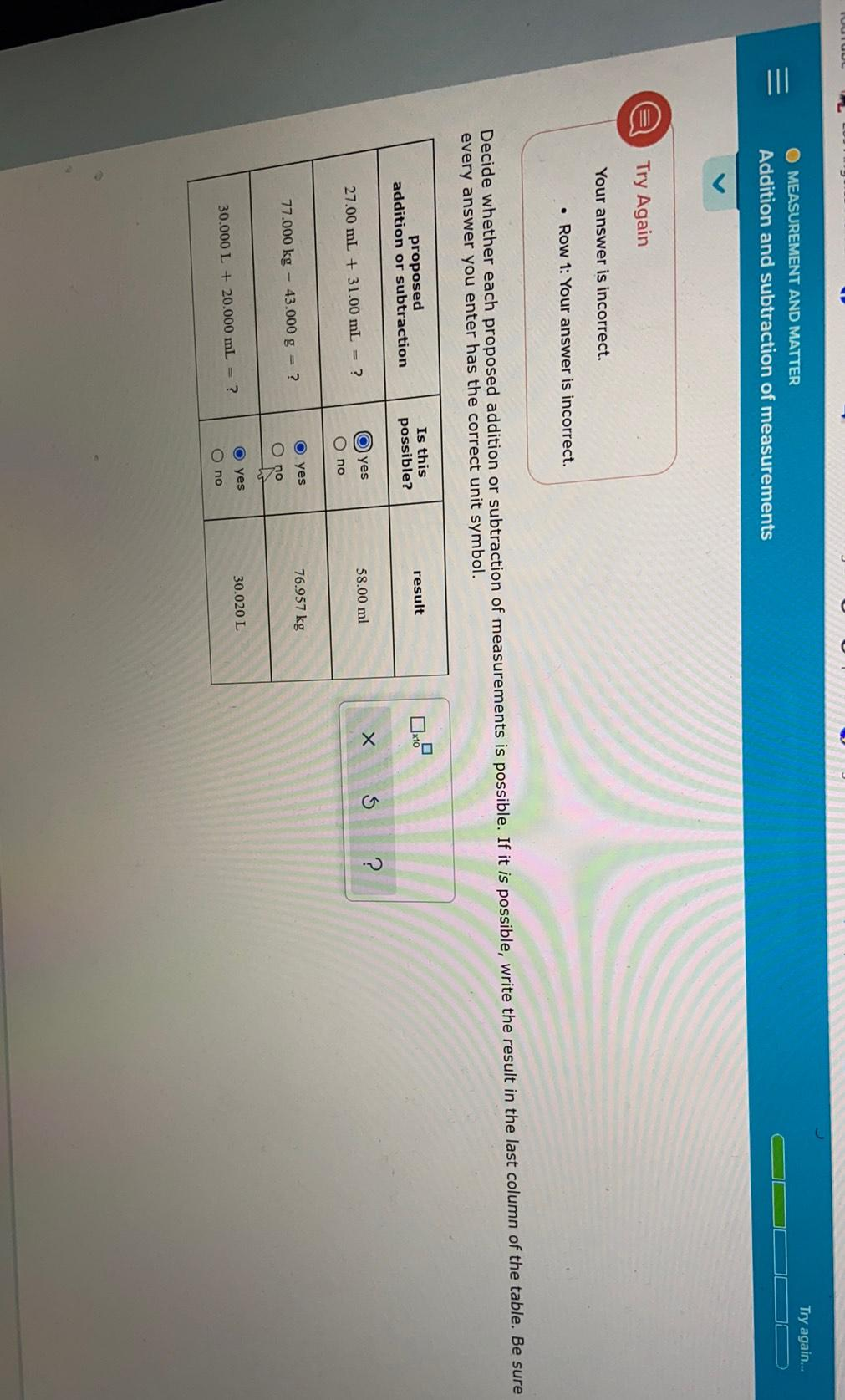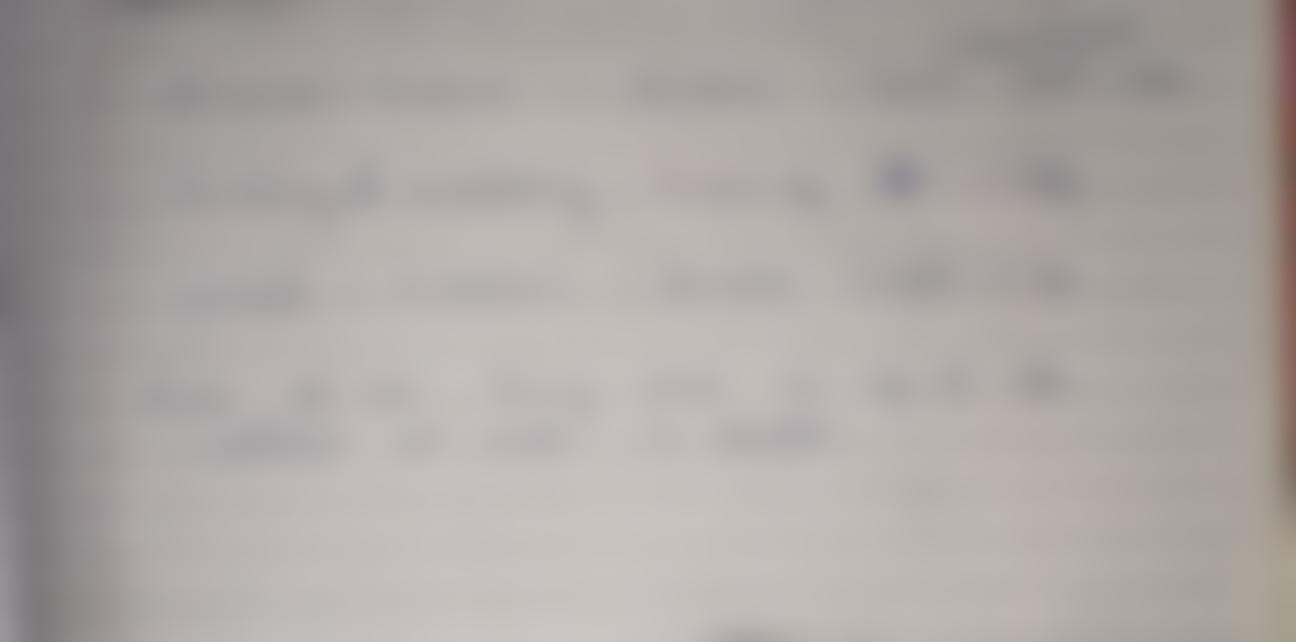Question:

Try again... O MEASUREMENT AND MATTER Addition and subtraction of measurements e Try Again Your answer is incorrect. • Row 1: YoTry again... O MEASUREMENT AND MATTER Addition and subtraction of measurements e Try Again Your answer is incorrect. • Row 1: Your answer is incorrect. Decide whether each proposed addition or subtraction of measurements is possible. If it is possible, write the result in the last column of the table. Be sure every answer you enter has the correct unit symbol. Is this possible? result proposed addition or subtraction x10 5 x ? 58.00 ml O) yes 27.00 mL + 31.00 ml = ? no 76.957 kg 77.000 kg - 43.000 g = ? O yes O no . yes 30.020 L 30.000 L + 20.000 mL = ? O no